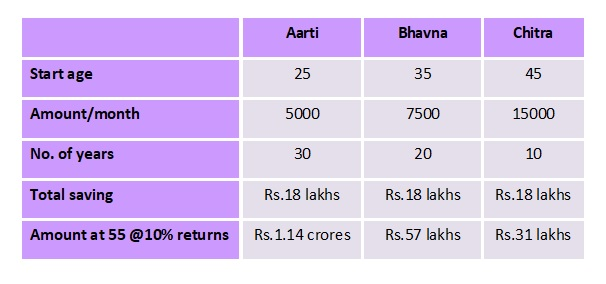# The Wealth Architects

(Precious) Words of Wisdom : "Never spend your money before you have earned it." ~ Thomas Jefferson

# How To Calculate Recurring Deposit Maturity Value In Excel

[This post is an update of the earlier blog posted on Sept 20, 2013]

Recurring Deposit (RD) is favoured by many investors who
(a) are able to save only small amounts every month,
(b) are looking for safe and assured returns and
(c) fall under the nil / lower income tax brackets.

Another good thing about RD is that there is NO painful TDS (Tax Deduction at Source) unlike on all other types of bank deposits. [Update: With effect from FY 2015-16, even recurring deposits will attract the provisions of TDS.

RD is very easy to understand and implement.

But the formula to calculate the maturity value is somewhat complicated. This is so because
...there is addition to the principal amount every month
...the interest paid by the banks in India is compounded quarterly.

However, Excel has made life quite simple.

You can, now, within a few minutes calculate how much money you will receive at maturity for a given recurring deposit.

Suppose you do a Recurring Deposit of Rs.500 for 36 months @8.75%. Then, the excel formula to calculate the amount receivable on maturity is as under:

= FV(Rate,Nper,Pmt,Pv,Type)

where
FV stands for Future Value
Rate = Modified Rate of interest  (I will come to "modified" later)
Nper = No. of deposits to be made (i.e. 36 in this example)
Pmt = Amount deposited every month (i.e. Rs.500 in this example)
Pv = Put this as 0 (PV stands for Present Value which is Zero)
Type = Put this as 1 as the deposit is made at the beginning of the month

Modified Rate of Interest: The interest rate in Recurring Deposits (in this case case of 8.75%) is compounded on quarterly basis. Whereas FV is calculated on monthly basis because we are making monthly deposits.So we cannot directly put the standard bank rate into the above formula.

We have to convert quarterly compounding rate into monthly compounding rate.

But don't worry, this is pretty simple.

Step 1: Convert the given quarterly compounding rate into Effective Annualized Rate
=EFFECT(Nominal_rate,Npery)
=EFFECT(8.75%,4)   [Npery is no. of times compounded i.e. 4 in this case]
=9.041%

Step 2: Convert the Effective Annualized Rate into monthly compounding rate
=NOMINAL(Effect_rate,Npery)
=NOMINAL(9.041%,12)    [Npery is no. of times compounded i.e 12 in this case]
=8.687%

Now we can apply the FV formula to work out the Maturity Value.
=FV(8.687%/12,36,500,0,1)
=Rs.20,627.38

[Note: Rate is divided by 12 as we are making monthly deposits]

That's it! Quick and simple.

(By the way, there is a still simpler method... you will come across many calculators online. But like me, for those who love to do things for themselves, the above calculations too are pretty straightforward.)

## An Investment In Knowledge Pays The Best Interest ~ Benjamin Franklin## Ignorance is like a SIGNED BLANK CHEQUE... anyone can MISUSE it.

Subscribe via Email

## ... Three VALUABLE Tips ...

 1. Which Is The Best Day To Invest Your Money?My "unique" take on the auspicious days to make the best investments. 2. Housewives Too Can Become MillionairesSaving for MORE TIME gives much better results than saving MORE MONEY. 3. Twist In Credit Card Interest Calculations You Must KnowCalculating Finance Charge on your Credit Card is LOT SIMPLER than this.